# ACT Math - Exponentials and Logarithms: Help and Review Chapter Exam

Exam Instructions:

Choose your answers to the questions and click 'Next' to see the next set of questions. You can skip questions if you would like and come back to them later with the yellow "Go To First Skipped Question" button. When you have completed the practice exam, a green submit button will appear. Click it to see your results. Good luck!

### Page 1

#### Question 2 2. Which logarithmic property is shown below?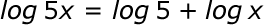#### Question 3 3. Evaluate the following.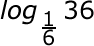#### Question 4 4. Solve the following equation for x.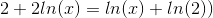#### Question 5 5. Simplify the following expression.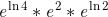### Page 2

#### Question 6 6. Solve the exponential equation below for x: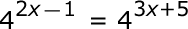#### Question 8 8. Evaluate the logarithm below to 2 decimal places.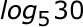#### Question 9 9. Evaluate the logarithm below: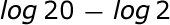#### Question 10 10. Solve, to 2 decimal places, the exponential equation below for x: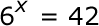### Page 3

#### Question 11 11. Use the following information to answer the question.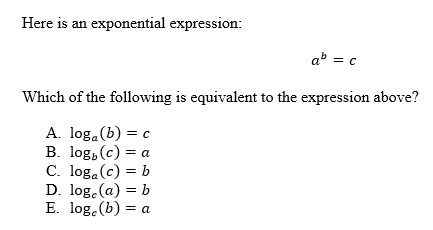#### Question 12 12. Solve the following equation for y = f(x).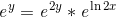#### Question 13 13. Expand the following logarithm: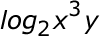#### Question 14 14. Condense the logarithm below: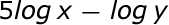### Page 4

#### Question 18 18. Evaluate the following.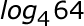#### Question 19 19. Evaluate the logarithm below: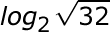#### Question 20 20. Solve the exponential equation below for x: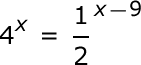### Page 5

#### Question 22 22. Evaluate the following.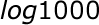### Page 6

#### Question 26 26. Solve the exponential equation below for x: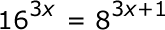#### Question 27 27. Simplify: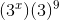#### ACT Math - Exponentials and Logarithms: Help and Review Chapter Exam Instructions

Choose your answers to the questions and click 'Next' to see the next set of questions. You can skip questions if you would like and come back to them later with the yellow "Go To First Skipped Question" button. When you have completed the practice exam, a green submit button will appear. Click it to see your results. Good luck!

Support